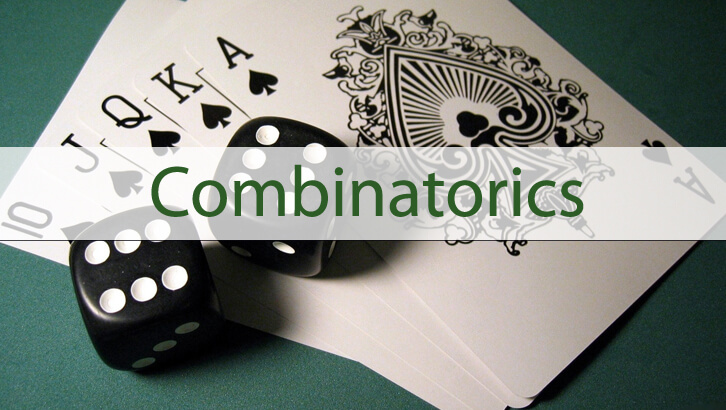# CAT Online Coaching – Permutation and Combination, Fixing the Errors III

This post had given a series of questions with incorrect solutions. Given below are the “debugged” solutions to questions 7, 8 and 9.

7. What is the probability of selecting 3 cards from a card pack such that all three are face cards? what is the probability that none of the three is a face card?
Given solution
Number of cards in a card pack = 52
Numbert of face cards in a card pack = 12
Number of ways of selecting 3 cards from a card pack = 52C3
Number of ways of selecting 3 face cards from a card pack = 12C3
Probability of selecting three cards such that all three are face cards = 12C3/52C3
Probability of selecting three cards such that none of the three are face cards = 1 – 12C3/52C3
Bug in the solution:
Other possibilities exist. As in, if we select three cards from a card pack, all three could be face cards, all three could be non-face cards, one could be a face card with 2 non-face cards or we could have two face cards and one non-face card. This is why we cannot use the 1 minus idea.
As a rule we can say P(A) = 1 – P(B) – P(C) if A, B and C are mutually exclusive and collectively exhaustive events. As in among them they should account for all possible events. And there should be no overlap. Creating a group of MECE equiprobable events is the most fundamentally brilliant idea in all of probability. Go on, look it up.
Correct solution:
This is simple. Probability of selecting three cards such that none of the three are face cards = 40C3/52C3
8. A die is rolled thrice. In how many outcomes will two throws be same and the third one different?
Given solution
Let the three outcomes be ABC.
‘A’ can take all values from 1 to 6
‘B’ can also take all values from 1 to 6
‘C’ can take all values except A – so it has 5 possibilities
Total number of outcomes = 6 * 6 * 5 = 180.
Bug in the solution:

The given solution is actually absurd. If two throws are to be same, then if A can take values from 1 to 6 and if B were equal to A, then b can take only one value. There can be no 6 * 6 * 5

Correct solution:
‘A’ can take all values from 1 to 6
‘B’ should be equal to A — One possibility
‘C’ can take all values except A – so it has 5 possibilities
6 * 1 * 5 = 30 outcomes.
There are 30 possible outcomes when A = B but not equal to C. Likewise, we could have A = C but not equal to B and B = C but not equal to A. So, there are totally 90 different possibilities.
9. How many 7 letter words can we have in English that have two distinct vowels and 5 distinct consonants.
Given solution
Now, we know there are 5 vowels and 21 onsonants. So, we need to select 2 from these 5 and 5 from the remaining 21. Since order is important, we need to select keeping order in mind.
So, we have 5P2 * 21P5.
Bug in the solution:
In this solution we do not account for intermingling of vowels and consonants. As in, we do not count words such as BACEDFG. We account for order within vowels and order within consonants, but we do not account for order across both categories.

Correct solution:
Select without accounting for order, then arrange everything put together
So, we have 5C2 * 21C5 * 7!.